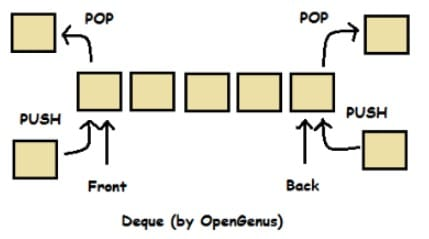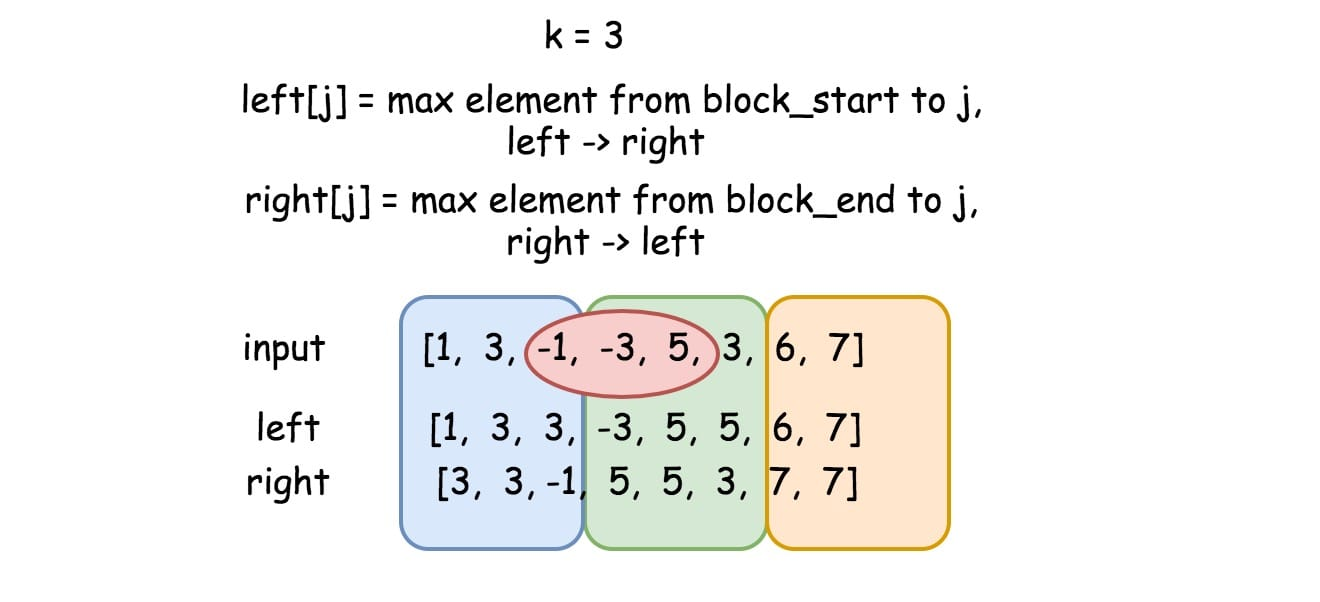# 239. Sliding Window Maximum

Reference: LeetCode
Difficulty: Hard

## Problem

Given an array nums, there is a sliding window of size k which is moving from the very left of the array to the very right. You can only see the k numbers in the window. Each time the sliding window moves right by one position. Return the max sliding window.

Note: You may assume k is always valid, 1 ≤ k ≤ input array’s size for non-empty array.

Example:

Follow up: Could you solve it in linear time?

## Analysis

### Brute-Force

Check every sliding window and compute the maximum value.

Time: $O(Nk)$
Space: $O(1)$ not including the output array

### Priority Queue

In theory, we can use priority queue to achieve $O(N\log{k})$. However, if we stick to the build-in PriorityQueue in Java, it still takes $O(Nk)$:

The code in indexOf() shows that this naive method takes $O(N)$ time in the worst case. Therefore, if we use the built-in method in Java, it takes $O(Nk)$.

In terms of $O(N\log{k})$, the basic idea is that we are keeping the size of the heap as at most k by removing the elements that are out of the k-size window. If we assume that remove() takes $O(\log{k})$ time (if we implement the priority queue by ourselves), it would have that logarithmic time complexity.

This is the first version I came up with. I think for this solution we can dive deeper to see how PriorityQueue in Java works. Consider what would happen if there are duplicates in the heap. Which one to be removed?

Also notice that when we are using the wrapper class Integer in Java we notice the caching feature.

This occurs because Java does caching for integers between -128 and 127 for better performance. Here is the code in Integer class:

Back to the problem. Therefore, if there are duplicates between -128 and 127 in the heap, we cannot delete the object that we want (the leftmost element in the sliding window).

But do duplicates actually matter? In other words, do we really need to consider the case where we have duplicate elements in the window and decide which one to remove?

The answer is NO. We can just remove one of them. For the example {2, 4, 2, 5}, k = 3, removing the first or the second 2 is the same.

However, we can modify the code a little bit and let the priority queue know the specific element we want to remove.

Time: $O(Nk)$ (if we implement PQ by ourselves, it is $O(N\log{k})$)
Space: $O(k)$

### Deque

If we can add and remove elements from both sides of the sliding window, we can solve this problem in linear time. It turns out that we can use Deque to achieve the goal. In the Deque, we add and remove indices.Basically, for each element nums[i] in the array that we are about to insert, we first check whether the leftmost index in the sliding window is out of bound. If so, we remove it by pollFirst() in constant time.

Then we continuously remove the rightmost indices if their corresponding elements are less than nums[i] (the element we are about to insert). The idea is that the elements that are less than the element we’ll insert won’t have any contributions to the maximum element of the sliding window. So it is safe to remove them.

After removal pollLast() and insertion offerLast(i) (the element nums[i]), we can say that the leftmost element in the window is maximum. Think about it why. Notice that the maximum element could be the one we just insert.

Last but not least, adding the maximum elements to the result array when necessary.

Time: $O(N)$ since each element is processed (add/remove) at most twice.
Space: $O(k)$

### DP

This method is very hacky. The explanation in solution section is readable.Note: Don’t read the code of my first attempt.

The better version:

Time: $O(N)$
Space: $O(N)$

CommentJunhao Wang
a software engineering cat